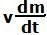## Thursday, June 12, 2008

### Irodov Solution 1.182To answer some questions and clarifications I decided to provide a bit more explanation to the solution of this problem. I will solve this problem using the basic Newton's laws .

Consider two instants of time t and t + dt, where dt is an infinitesimally small interval of time. Suppose that the mass of the cart at time t was m and that at time t + dt was m-dm. Further suppose that the speed of the cart at time t was v and that at t + dt was v + dv.

The momentum of the cart at time t was mv. The momentum of the cart at time t+dt was (m-dm)(v+dv). Now the dm mass of sand that left the cart in the dt interval would be still moving in the horizontal direction with a speed v. Hence the momentum of the sand that fell off the cart is given by vdm. The total momentum of the (sand + cart) system at time t+dt is sum of the momentum of the cart and the momentum of the sand that fell off which is given by (m-dm)(v+dv) + vdm. The change in momentum of the (cart + sand) system due to the force on the (cart + sand) system during this interval is given by Fdt. Hence, we have,Basically, as the sand falls out, the force F has to pull a progressively lighter cart and so the acceleration of the cart increases with time. The mass of the cart varies as. So we have,Some students have asked as to why we do not see theterm as we saw in problem 1.181. I hope that the reason is clear from the initial explanation above. Basically, the force F does not act on the dm part of sand that has left the cart and so its momentum remains intact. When unsure please use basic principles and you will not go wrong.